Start typing, then use the up and down arrows to select an option from the list.# Molarity Example 1

Jules Bruno
295views
5
1
here, it says, to calculate the molar ity of a solution prepared by dissolving 23. g of sodium hydroxide in enough water to make 2. liters of solution. So here we're looking for more clarity. So mole arat E. Here equals are moles of solute, which would be the H. And it is. It's in a solution off 2.0 leaders, so we need leaders off solution. All right, we already have the 2.50 liters of solution, so we just need to convert the grams of sodium hydroxide into moles. So one mole of sodium hydroxide goes on the bottom, and then grams of sodium hydroxide goal. One more goes on the top and grams of sodium hydroxide go on the bottom. Sodium hydroxide is composed off one sodium, one oxygen and one hydrogen. Multiply them by their atomic masses from the periodic table, okay and then add up the totals. This will give us the Moller mask of sodium hydroxide, so we add that all up, it's 39.998 g, so that's going to go here. So here grams of sodium hydroxide cancel out and Now I'm gonna have moles of sodium hydroxide. So that comes out 2.59 to 5 moles. Take that and plug it in and we'll have the mole polarity of our solution. So this comes out 2. Moeller for solution.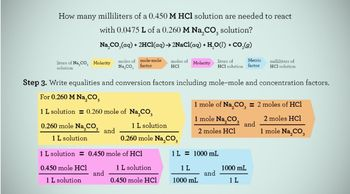08:09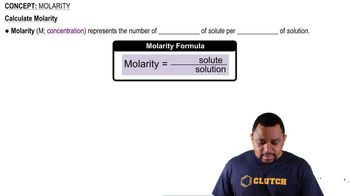00:53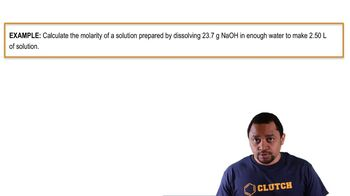01:46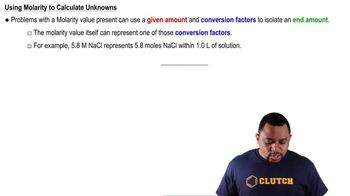01:13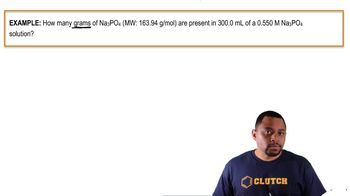03:47